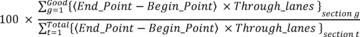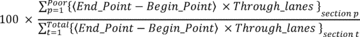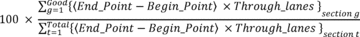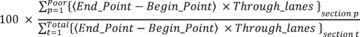Title 23

SECTION 490.313

490.313 Calculation of performance management measures.

§ 490.313 Calculation of performance management measures.

(a) The pavement measures in § 490.307 shall be calculated in accordance with this section and used by State DOTs and MPOs to carry out the pavement condition related requirements of this part, and by FHWA to make the significant progress and minimum condition determinations specified in §§ 490.109 and 490.317, respectively.

(b) The performance measure for pavements shall be calculated based on the data collected in § 490.309 and pavement condition metrics computed in § 490.311. The performance measure for pavements shall be based on three condition ratings of Good, Fair, and Poor calculated for each pavement section. The ratings are determined as follows:

(1) IRI rating shall be determined for all pavement types using the following criteria. If an IRI value of a pavement section is: -

(i) Less than 95, the IRI rating for the pavement section is Good;

(ii) Between 95 and 170, the IRI rating for the pavement section is Fair; and

(iii) Greater than 170, the IRI rating for the pavement section is Poor.

(2) Cracking condition shall be determined using the following criteria:

(i) For asphalt pavement sections -

(A) If the Cracking_Percent value of a section is less than 5 percent, the cracking rating for the pavement section is Good;

(B) If the Cracking_Percent value of a section is equal to or greater than 5 percent and less than or equal to 20 percent the cracking rating for the pavement section is Fair; and

(C) If the Cracking_Percent value of a section is greater than 20 percent the cracking rating for the pavement section is Poor.

(ii) For jointed concrete pavement sections -

(A) If the Cracking_Percent value of a section is less than 5 percent, the cracking rating for the pavement section is Good;

(B) If the Cracking_Percent value of a section is equal to or greater than 5 percent and less than or equal to 15 percent the cracking rating for the pavement section is Fair; and

(C) If the Cracking_Percent value of a section is greater than 15 percent the cracking rating for the pavement section is Poor.

(iii) For CRCP sections:

(A) If the Cracking_Percent value of a section is less than 5 percent, the cracking rating for the pavement section is Good;

(B) If the Cracking_Percent value of a section is equal to or greater than 5 percent and less than or equal to 10 percent, the cracking rating for the pavement section is Fair; and

(C) If the Cracking_Percent value of a section is greater than 10 percent, the cracking rating for the pavement section is Poor.

(3) Rutting or faulting rating shall be determined using the following criteria.

(i) For asphalt pavement:

(A) If the rutting value of a section is less than 0.20 inches, the rutting rating for the pavement section is Good;

(B) If the rutting value of a section is equal to or greater than 0.20 inches and less than or equal to 0.40 inches, the rutting rating for the pavement section is Fair; and

(C) If the rutting value of a section in is greater than 0.40 inches, the rutting rating for the pavement section is Poor.

(ii) For jointed concrete pavement:

(A) If the faulting value of a section is less than 0.10 inches, the faulting rating for the pavement section is Good;

(B) If the faulting value of a section is equal to or greater than 0.10 inches and less than or equal to 0.15 inches, the faulting rating for the pavement section is Fair; and

(C) If the faulting value of a section is greater than 0.15 inches, the faulting rating for the pavement section is Poor.

(4) The FHWA will determine that a reported section in HPMS has a missing, invalid or unresolved data on the dates specified in § 490.317(b) for Interstate System and § 490.109(d)(2) and (d)(4) for non-Interstate NHS, if a reported section does not meet any one of the data requirements specified in §§ 490.309 and 490.311(c) or that reported section does not provide sufficient data to determine its Overall Condition specified in paragraphs (c) through (f) of this section:

(i) Total mainline lane-miles of missing, invalid, or unresolved sections for Interstate System and non-Interstate NHS shall be limited to no more than 5 percent of the total lane miles less the sections excluded in § 490.313(f)(1). For each pavement section without collected its condition metrics and inventory data, State DOTs shall note in the HPMS submittal with a specific code identified in the HPMS Field Manual (incorporated by reference, see § 490.111) noting the reason it was not collected.

(ii) Calculation of overall pavement conditions in any State meeting the requirements of § 490.309(b) shall be based only on sections containing data reported in the HPMS Submittal as of the submission dates required in § 490.311(c)(4) and (5). State DOTs not meeting the requirements of § 490.309(b) will be considered as not in compliance with § 420.105(b) requiring State DOTs to submit data to the HPMS and not in compliance with § 490.107 requiring reporting on performance targets. Failure to report data meeting the requirements of § 490.309(b) by the submission dates for the Interstate System will be considered as not meeting the minimum requirements for pavement conditions on the Interstate System and that State DOT is subject to the penalties in § 490.315.

(c) The Overall condition for asphalt and jointed concrete pavement sections shall be determined based on the ratings for IRI, Cracking_Percent, rutting and faulting, as described in paragraphs (b)(1), (b)(2), (b)(3) and (b)(4) of this section, respectively, for each section as follows:

(1) A pavement section shall be rated an overall condition of Good only if the section is exhibiting Good ratings for all three conditions (IRI, Cracking_Percent, and rutting or faulting);

(2) A pavement section shall be rated an overall condition of Poor if two or more of the three conditions are exhibiting Poor ratings (at least two ratings of Poor for IRI, Cracking_Percent, and rutting or faulting).

(3) A pavement section shall be rated an overall condition of Fair if it does not meet the criteria in paragraphs (c)(1) or (c)(2) of this section.

(4) For sections on roadways where the posted speed limit is less than 40 MPH and where the State DOT has reported PSR in lieu of the IRI, Cracking_Percent, rutting, and faulting metrics the PSR condition level shall be determined using the following criteria:

(i) If the PSR of a section is equal to or greater than 4.0 the PSR rating for the pavement section is Good;

(ii) If the PSR of a section is less than 4.0 and greater than 2.0 the PSR rating for the pavement section is Fair; and

(iii) If the PSR of a section is less than or equal to 2.0 the PSR rating for the pavement section is Poor.

(d) The Overall condition for CRCP sections shall be determined based on two ratings of IRI and Cracking_Percent, as described in paragraphs (b)(1) and (b)(2) of this section or based on PSR where appropriate as described in paragraph (c)(4) of this section, respectively, for each section as follows:

(1) A pavement section shall be rated an overall condition of Good only if the section is exhibiting Good ratings for both conditions (IRI and Cracking_Percent);

(2) A pavement section shall be rated an overall condition of Poor if it exhibits Poor ratings for both conditions (IRI and Cracking_Percent);

(3) A pavement section shall be rated an overall condition of Fair if it does not meet the criteria in paragraphs (d)(1) or (d)(2) of this section.

(4) For pavement sections that are on roadways with a posted speed limit of less than 40 MPH where the State DOT reported the PSR metric in lieu of the IRI, Cracking_Percent, faulting, and rutting metrics the pavement section shall be rated an overall condition equal to the PSR condition rating as described in section (c)(4) above

(e) State DOTs shall not be subject to paragraphs (c) and (d) of this section for Pavements on the until after the data collection cycle ending December 31, 2018, for Interstate highways and December 31, 2021, for the non-Interstate NHS. During this transition period, the Overall condition for all pavement types will be based on IRI rating, as described in paragraph (b)(1) of this section, or on PSR as described in paragraphs (c)(4) or (d)(4) of this section.

(f) The pavement condition measures in § 490.307 shall be computed as described below. The measures shall be used for establishing targets in accordance with § 490.105 and reporting the conditions of the pavements in the biennial performance reporting required in § 490.107 as follows:

(1) Bridges shall be excluded prior to computing all pavement condition measures by removing the sections where the Structure_Type data item in the HPMS is coded as 1. Sections that have an unpaved surface or an “other” surface type (such as cobblestone, planks, brick) shall be excluded prior to computing all pavement condition measures by removing the sections where the Surface Type data item in the HPMS is coded as 1 or as 11.

(2) For § 490.307(a)(1) the measure for percentage of lane-miles of the Interstate System in Good condition shall be computed to the one tenth of a percent as follows:Where: Good = total number of mainline highway Interstate System sections where the overall condition is Good; g = a section's overall condition is determined Good per paragraphs (b) or (c) of this section; t = an Interstate System section; Total = total number of mainline highway Interstate System sections excluding bridges, unpaved surface and “other” surface types, and missing data sections, described in paragraph (f)(1) and (b)(4)(i) of this section. Begin_Point = Begin Milepost of each section g or t; End Point = End Milepost of each section g or t; and Through_lanes = the number of lanes designated for through-traffic represented by a section g or t.

(3) For § 490.307(a)(2) the measure for percentage of lane-miles of the Interstate System in Poor condition shall be computed to the one tenth of a percent as follows:Where: Poor = total number of mainline highway Interstate System sections where the overall condition is Poor; p = a section's overall condition is determined Poor per paragraphs (b) or (c) of this section; t = an Interstate System section; Total = total number of mainline highway Interstate System sections excluding bridges, unpaved surface and “other” surface types, and missing data sections, described in paragraph (f)(1) and (b)(4)(i) of this section; Begin_Point = Begin Milepost of each section p or t; End Point = End Milepost of each section p or t; and Through_lanes = the number of lanes designated for through-traffic represented by a section p or t.

(4) For § 490.307(a)(3) the measure for percentage of lane-miles of the non-Interstate NHS in Good condition in § 490.307(a)(3) shall be computed to the one tenth of a percent as follows:Where: Good = total number of mainline highway non-Interstate NHS sections where the overall condition is Good; g = a section's overall condition is determined Good per paragraphs (b), (c) or (d) of this section; t = a non-Interstate NHS section; Total = total number of mainline highway non-Interstate NHS sections excluding bridges, unpaved surface and “other” surface types, and missing data sections, described in paragraph (f)(1) and (b)(4)(i) of this section; Begin_Point = Begin Milepost of each section g or t; End Point = End Milepost of each section g or t; and Through_lanes = the number of lanes designated for through-traffic represented by a section g or t.

(5) For § 490.307(a)(4) the measure for percentage of lane-miles of the non-Interstate NHS in Poor condition in § 490.307(a)(4) shall be computed to the one tenth of a percent as follows:Where: Poor = total number of mainline highway non-Interstate NHS sections where the overall condition is Poor; p = a section's overall condition is determined Poor per paragraphs (b), (c) or (d) of this section; t = a non-Interstate NHS section; Total = total number of mainline highway non-Interstate NHS sections excluding bridges, unpaved surface and “other” surface types, and missing data sections, described in paragraph (f)(1) and (b)(4)(i) of this section; Begin_Point = Begin Milepost of each section p or t; End Point = End Milepost of each section p or t; and Through_lanes = the number of lanes designated for through-traffic represented by a section p or t.